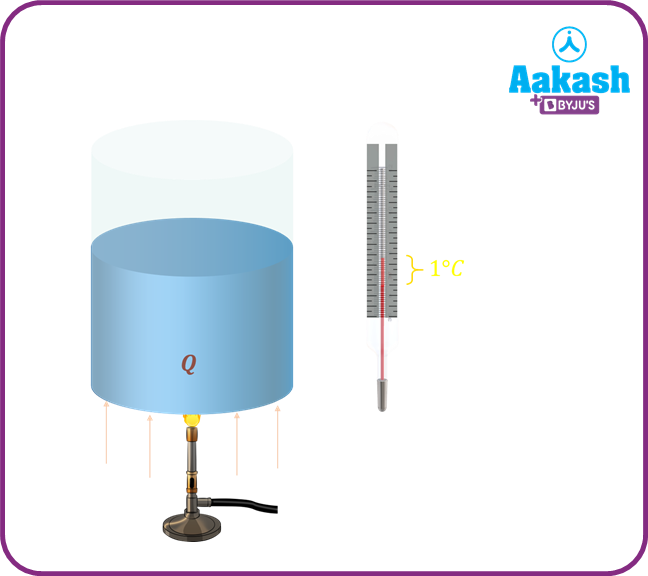• Call Now

1800-102-2727•

# Calorimeter - Definition, Diagrams, Practice problems, FAQs

Nitin makes a cup of hot coffee in the morning. The cup of coffee is so hot that vapors are seen coming out from it. He then drops a piece of ice cube into a cup containing boiling coffee. He notices that the ice cube dissolves fast. He drops one more cube, and notices that the same thing happens again. Also, the vapors coming from the coffee have subsided. This is proof that coffee has cooled down. On the other hand, the ice cube would have gained heat. The same happens when two liquids having uneven temperatures are mixed; the hotter liquid becomes colder, and the colder liquid becomes hotter. Study of such heat lost or gained is calorimetry. In this article, we will explore calorimetry in detail.

• Principle of calorimetry
• Specific heat capacity
• Latent heat of fusion
• Examples of calorimetry
• Practice problems
• FAQs

## Principle of calorimetry

Calorimetry deals with measurement of heat. When two bodies, having different temperatures, are placed in contact with each other, heat flows from the body having higher temperature to the one having lower temperature. Hence, the heat lost by the hotter body is equal to the heat lost by the cold body. This is in accordance with the law of conservation of energy. An apparatus used to measure the heat changes occuring in a reaction is called bomb calorimeter.

## Specific heat capacity

The amount of heat required to raise the temperature of 1 kg of a substance by unity (10C or 1K) is called specific heat capacity(C). If $\Delta \theta$ be the change in temperature, and Q be the amount of heat supplied to it. Then$Q=mC\Delta \theta$; m- mass of the substance being heated.

The unit of specific heat capacity is $\frac{\frac{J}{kg}}{K}$ or $\frac{J}{kg{\frac{}{}}^{0}C}.$. Its dimensional formula is $\left[{M}^{0}{L}^{2}{T}^{-2}{\theta }^{-1}\right].$

## Latent heat of fusion

When a substance undergoes a phase change, then some amount of heat is lost or gained. For instance, when ice melts to form water, it is an endothermic reaction, meaning heat is absorbed in it. On the other hand, when water freezes to form ice, heat is released. Let Q be the amount of heat released or absorbed, and L be the latent heat of fusion.Then,

Q=mL.

The CGS unit of latent heat of fusion is $\frac{cal}{g}$ and its SI unit is $Jk{g}^{-1}$. Its dimensional formula is

Lv- latent heat of fusion of steam$=540\frac{cal}{g}$

Lice- latent heat of fusion of ice$=80\frac{cal}{g}$

Examples of calorimetry

Consider m1 kg of steam at 1000C being mixed with m2 kg of water at 300C. If T indicates the temperature of the mixture, then

Heat lost by steam=heat gained by water

${m}_{1}{L}_{v}+{m}_{1}{C}_{1}\left(100-T\right)={m}_{2}{C}_{2}\left(T-30\right)$

C2- specific heat capacity of water

C1- specific heat capacity of steam

Consider m1 kg of ice at 00C being mixed with m2 kg of water at 400C. If T indicates the common temperature of the mixture after mixing, then

Heat gained by ice=heat lost by water

C2- specific heat capacity of water.

C3- specific heat capacity of ice.

## Practice problems

Q1.Calculate the energy gained when 5 kg of water at 200C is heated to its boiling point?(given, specific heat of water$=4.2\frac{kJ}{kg{\frac{}{}}^{0}C}.$

Answer. Given, m=5 kg, $\Delta \theta ={100}^{0}C-{20}^{0}C={80}^{0}C.$

$C=4200\frac{J}{kg{\frac{}{}}^{0}C}.$

Q2.100 kg of water at 800 C is mixed with 300 kg of water at 600 C. The final temperature reached by the mixture would be

(a)700C                                                         (b)650C
(c)600C                                                        (d)750C

Let T indicate the final temperature of the mixture.

$100×{S}_{water}×\left(80-T\right)=300×{S}_{water}×\left(T-60\right)$

Q3. 50g of ice at 00 C is mixed with 50 g of water at 600 C, final temperature of the mixture would be

When 50 g of water at 600C mixes with 50 g of ice at 00 C.

Heat lost by water,

Q2- heat absorbed by ice. Let m' be the mass of the ice.

${Q}_{2}={m}^{\text{'}{L}_{ice}}$;${L}_{ice}=80\frac{cal}{g}$

all of the ice has not melted. Hence the final temperature of the mixture would be 00C.

Q4. 100 grams of ice, initially at 00C is mixed with 100 g of water kept at 1000 C. Find the final temperature of the mixture.

(a)100C                                      (b)200C
(c)300C                                     (d)00C

Heat lost by ice=Heat gained by water

$100×80+100×1×\left(T-0\right)=100×1×\left(100-T\right)$

$T={10}^{0}C.$

Q4.Calculate the heat capacity of 40 g of aluminum. (Specific heat capacity of aluminum$C=0.2\frac{cal}{g{\frac{}{}}^{0}C}$

(a)                                                   (b)
(c)                                                 (d)

Mass of the aluminum m=40 g, Specific heat of aluminum $C=0.2\frac{cal}{g{\frac{}{}}^{0}C}$

Heat capacity

## FAQs

Q1.What are some limitations of calorimetry?
If proper insulation is not provided, the bomb calorimeter loses heat to the surroundings. Also, uneven heating occurs if the mixture is not well mixed. Explosion of substances like TNT cannot be contained in a calorimeter.

Q2.On what principle does a calorimeter operate?